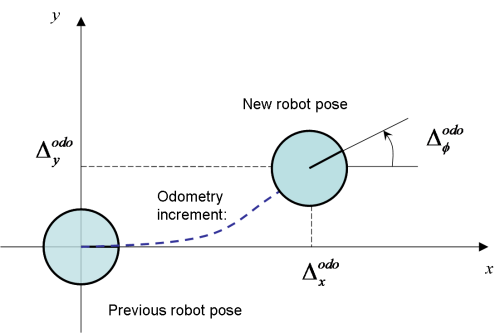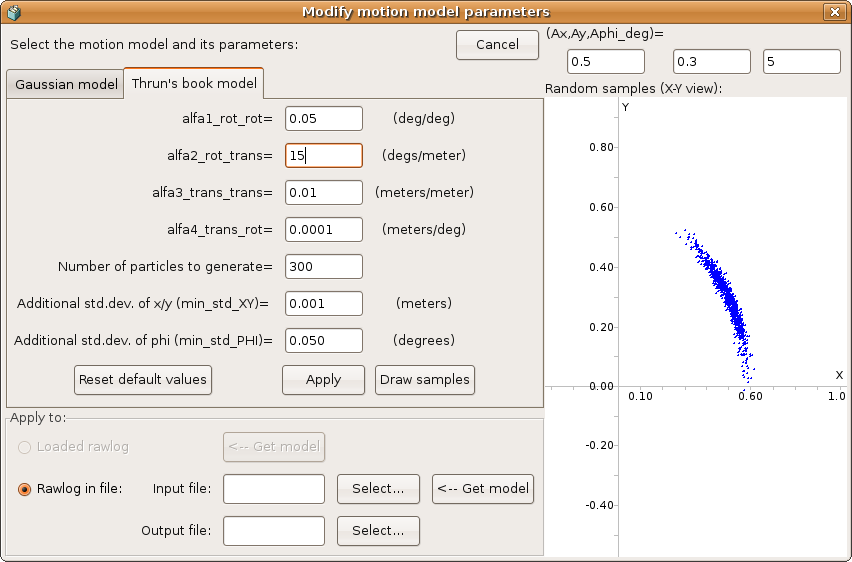# Probabilistic Motion Models

## 1. Introduction

Within a particle filter, the samples are propagated at each time step using some given proposal distribution. A common approach
for mobile robots is taking the probabilistic motion model directly as this proposal.

In the MRPT there are two models for probabilistic 2D motion, implemented in mrpt::slam::CActionRobotMovement2D. To use them just fill out the option structure “motionModelConfiguration” and select the method in “CActionRobotMovement2D::TMotionModelOptions::modelSelection”.

An example of usage would be like:

This page provides a description of the internal workings of these methods.

## 2. Gaussian probabilistic motion model

Assume the odometry is read as incremental changes in the 2D robot pose. The odometry readings are denoted as$\left( \Delta^{odo}_x ~ \Delta^{odo}_y ~ \Delta^{odo}_\phi \right)$

The model for these variables is:The equations that relate the prior robot pose$\left( x ~ y ~ \phi \right)$ and the new pose$\left( x' ~ y' ~ \phi' \right)$ after the incremental change are: (based on the proposal in )$\left( \begin{array}{c} x' \\ y' \\ \phi' \end{array} \right) = \left( \begin{array}{c} x \\ y \\ \phi \end{array} \right) + \left( \begin{array}{ccc} \cos(\phi+\frac{\Delta^{odo}_\phi}{2}) ~ & ~ -\sin(\phi+\frac{\Delta^{odo}_\phi}{2}) ~ & ~ 0 ~ \\ \sin(\phi+\frac{\Delta^{odo}_\phi}{2}) ~ & ~ \cos(\phi+\frac{\Delta^{odo}_\phi}{2}) ~ & ~ 0 ~ \\ 0 ~ & ~ 0 ~ & ~ 1 \end{array} \right) \left( \begin{array}{c} \Delta^{odo}_x \\ \Delta^{odo}_y \\ \Delta^{odo}_\phi \end{array} \right)$

Our aim here is to obtain a multivariate Gaussian distribution of the new pose, given that the prior pose has a known value (it is the particle being propragated). In this case we can just model how to draw samples from a prior pose of$(0~0~0)$, and then the samples can be composed using the actual prior pose.

Using this simplification:$\left( \begin{array}{c} x' \\ y' \\ \phi' \end{array} \right) = \left( \begin{array}{ccc} \cos \frac{\Delta^{odo}_\phi}{2} ~ & ~ -\sin \frac{\Delta^{odo}_\phi}{2} ~ & ~ 0 ~ \\ \sin \frac{\Delta^{odo}_\phi}{2} ~ & ~ \cos \frac{\Delta^{odo}_\phi}{2} ~ & ~ 0 ~ \\ 0 ~ & ~ 0 ~ & ~ 1 \end{array} \right) \left( \begin{array}{c} \Delta^{odo}_x \\ \Delta^{odo}_y \\ \Delta^{odo}_\phi \end{array} \right) = H \left( \begin{array}{c} \Delta^{odo}_x \\ \Delta^{odo}_y \\ \Delta^{odo}_\phi \end{array} \right)$

The mean of the Gaussian can be simply computed from the composition of the prior and the odometry increment. For the covariance, we need to estimate the variances of the three variables of the odometry increment. We model them as having independent, zero-mean Gaussian errors. The errors will be composed of terms that capture imperfect odometry and potential drift effects.

We denote as$\Sigma$ the diagonal matrix having the three variances of the odometry variables, modeled as:$\begin{array}{l} \sigma_{\Delta^{odo}_x} = \sigma_{\Delta^{odo}_y} = \sigma^{min}_{xy} + \alpha_1 \sqrt{ (\Delta^{odo}_x)^2 + (\Delta^{odo}_y)^2} + \alpha_2 | \Delta^{odo}_\phi | \\ \sigma_{\Delta^{odo}_\phi} = \sigma^{min}_{\phi} + \alpha_3 \sqrt{ (\Delta^{odo}_x)^2 + (\Delta^{odo}_y)^2} + \alpha_4 | \Delta^{odo}_\phi | \end{array}$

The default parameters (loaded in the constructor and available in RawLogViewer) are:$\begin{array}{cc} \alpha_1 =& 0.05 ~ meters/meter \\ \alpha_2 =& 0.001 ~ meters/degree \\ \alpha_3 =& 5 ~ degrees/meter \\ \alpha_4 =& 0.05 ~ degrees/degree \\ \sigma^{min}_{xy} =& 0.01 ~ meters \\ \sigma^{min}_{\phi} =& 0.20 ~ degrees \end{array}$

And finally, the covariance of the new pose after the odometry increment ($C$) is computed by means of:$C = J ~ \Sigma ~ J^t$
where J stands for the Jacobian of H. See  for a derivation of this formula for error propagation.

The following is an example of samples obtained using this model with the RawLogViewer application:## 3. Thrun, Fox & Burgard’s book particle motion model

Like above, denote the odometry readings as$\left( \Delta^{odo}_x ~ \Delta^{odo}_y ~ \Delta^{odo}_\phi \right)$, and let’s assume that the prior robot pose is$(0~0~0)$, which means that we want to draw samples of the robot increment, not the final robot pose (to simplify the equations without loss of generality). Then, the new robot pose, which we want to draw samples from is:$\left( \begin{array}{c} x' \\ y' \\ \phi' \end{array} \right) = \left( \begin{array}{ccc} \cos \hat\delta_{rot1} ~ & ~ 0 ~ & ~ 0 ~ \\ \sin \hat\delta_{rot1} ~ & ~ 0 ~ & ~ 0 ~ \\ 0 ~ & ~ 1 ~ & ~ 1 \end{array} \right) \left( \begin{array}{c} \hat\delta_{trans} \\ \hat\delta_{rot1} \\ \hat\delta_{rot2} \end{array} \right)$

Where the variables correspond to the robot pose increment as is shown in the figure:

Here, the variables$\hat\delta_{trans}$,$\hat\delta_{rot1}$ and$\hat\delta_{rot2}$ are the result of adding a Gaussian, zero-mean random noise to the actual odometry readings:$\begin{array}{cc} \hat\delta_{trans} =& \delta_{trans} + \epsilon_{trans} ~~~~~~~~ \epsilon_{trans} \sim \mathcal{N}(0, \sigma^2_{trans}) \\ \hat\delta_{rot1} =& \delta_{rot1} + \epsilon_{rot1} ~~~~~~~~ \epsilon_{rot1} \sim \mathcal{N}(0, \sigma^2_{rot1}) \\ \hat\delta_{rot2} =& \delta_{rot2} + \epsilon_{rot2} ~~~~~~~~ \epsilon_{rot2} \sim \mathcal{N}(0, \sigma^2_{rot2}) \end{array}$

The model described in  employs the following approximations for the values of the standard deviations required for the equations above:$\begin{array}{rl} \sigma_{rot1} =& \alpha_1 |\delta_{rot1}| + \alpha_2 \delta_{trans} \\ \sigma_{trans} =& \alpha_3 \delta_{trans} + \alpha_4 ( |\delta_{rot1}| + |\delta_{rot2}| ) \\ \sigma_{rot2} =& \alpha_1 |\delta_{rot2}| + \alpha_2 \delta_{trans} \end{array}$

This is the model implemented in CActionRobotMovement2D when setting “CActionRobotMovement2D::TMotionModelOptions::modelSelection” to “mmThrun”. Actually, a small additional error is summed to each pose component ($x,y,\phi$) to avoid that for a null odometry increment the movement for all the particles become exactly zero, which may lead a particle filter to degenerate.

Below it is shown an example of samples generated using this model, for an excessively large value of$\alpha_2$ (a very large “slippage”), generated by RawLogViewer: